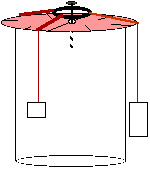# Physics

#### Physics Lab: Force TablePhysics Lab

# Physics Lab: Force Table

## Goal: to become familiar with force measurements.

#### Materials and Equipment

Remember that the setup below is a suggestion; any setup which will produce a force table on which you can read the angles of your as-much-as-possible freely swinging weights will work.

• a metal ring (use frictionless ring from kit if you have it)
• nail
• nylon thread (must be smooth)
• two half-moon protractors made of clear plastic
• stand (for example, a tall thin box, such as an oatmeal carton, with a flat top)
• standard masses (such as nickels, at 5 gr each)#### Procedure

Assemble the force table as in the diagram. You may need to invert one of the protractors, so be careful when you are reading angles.

1. The biggest source of error in this system will be due to friction where the string touches the table surface, so if you can think of a way to minimize friction there, do so. Physics lab force tables use pulleys for each string; any curved surface will help.
2. Decide which half is the 0°-180° half of the circle, and which is the 180°-360° portion.
3. Tie four nylon strings to the ring. These should be long enough to hang over the edge of your table and allow the attached weights to swing freely.
4. Using three or four weights of differing masses, stabilize the ring around the nail, so that it is centered but not touching the nail.
5. Record your hypothesis: is the sum of the forces in this situation <0, =0, or >0?
6. Record the masses on each string, and the location (0°- 360°) around the table.
7. Draw a force vector diagram of your system in equilibrium.
8. Sum all the horizontal forces.
9. Sum all the vertical forces.
10. What do you expect to get for the sum of all the forces in this situation?
11. Estimate errors for all measurements and discuss their effect on in your measurements.
12. Explain any deviations in experimental data from your calculated data.
13. Repeat this procedure for at least 4 different combinations of masses and positions.